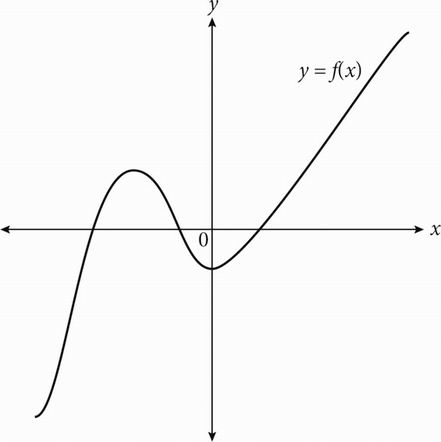# SAT Math Multiple Choice Question 208: Answer and Explanation

### Test Information

Question: 208

13.The figure above shows the graph in the xy-plane of the function f. If q, r, s and t are distinct real numbers, which of the following could be f(x) ?

• A. f(x) = (x - q)2
• B. f(x) = (x - r)(x + s)
• C. f(x) = (x - r)(x + s)(x + t)
• D. f(x) = (x - q)(x - r)(x + s)(x + t)